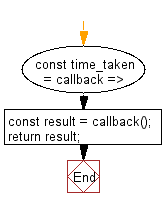# JavaScript: Measure the time taken by a function to execute

## JavaScript fundamental (ES6 Syntax): Exercise-28 with Solution

Write a JavaScript program to measure the time taken by a function to execute.

• Use Console.time() and Console.timeEnd() to measure the difference between the start and end times to determine how long the callback took to execute.

Sample Solution:

JavaScript Code:

``````const time_taken = callback => {
const result = callback();
return result;
};
console.log("Time taken: " + time_taken(() => Math.pow(2, 10))+" ms");
console.log("Time taken: " + time_taken(() => Math.sqrt(225))+" ms");
console.log("Time taken: " + time_taken(() => Math.sqrt((5 * 5) + (6 * 6)))+" ms");
```
```

Sample Output:

```Time taken: 1024 ms
Time taken: 15 ms
Time taken: 7.810249675906654 ms
```

Flowchart:Note : The code above is written in ES6 and can be executed as intended on Chrome Developer Tools.

Improve this sample solution and post your code through Disqus

What is the difficulty level of this exercise?

Test your Programming skills with w3resource's quiz.

﻿

## JavaScript: Tips of the Day

Chunks an array into n smaller arrays

Example:

```const tips_chunkIntoN = (arr, n) => {
const size = Math.ceil(arr.length / n);
return Array.from({ length: n }, (v, i) =>
arr.slice(i * size, i * size + size)
);
}
console.log(tips_chunkIntoN([1, 2, 3, 4, 5, 6, 7,8], 4));
```

Output:

```[[1,2],[3,4],[5,6],[7,8]]
```